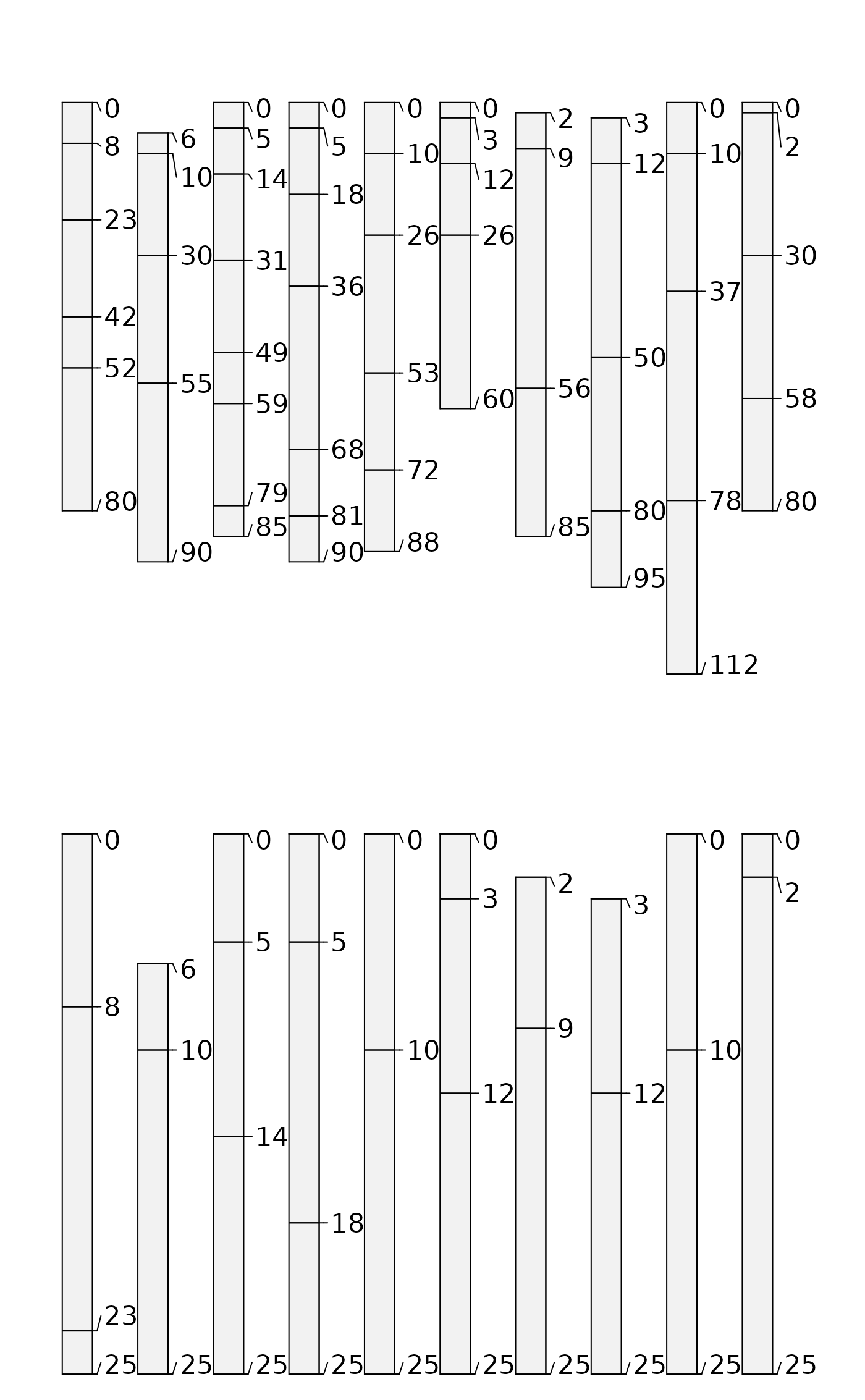Make a "clod" of horizons from a SoilProfileCollection given a point or a depth interval to intersect. The interval [z1,z2] may be profile-specific (equal in length to p), or may be recycled over all profiles (if boundaries are length 1). For "point" intersection, z2 may be left as the default value NULL.

trunc() is a wrapper method (using S4 generic) for glom() where truncate=TRUE

# S4 method for SoilProfileCollection
glom(
p,
z1,
z2 = NULL,
ids = FALSE,
df = FALSE,
truncate = FALSE,
invert = FALSE,
fill = FALSE,
modality = "all",
drop = !fill,
...
)

# S4 method for SoilProfileCollection
trunc(x, z1, z2, ...)

## Arguments

p

A SoilProfileCollection

z1

numeric vector of top depth to intersect horizon (required). Can be an expression involving siteNames(p) or quoted column name. Should evaluate to numeric length 1 or length equal to length(p)

z2

numeric vector bottom depth of intersection interval (optional). Can also be an expression involving siteNames(p) or quoted column name. Should evaluate to numeric length 1, length equal to length(p) or NULL. Default: NULL is "point" intersection

ids

return only horizon IDs? default: FALSE

df

return a data.frame, by intersection with horizons(p)? default: FALSE

truncate

truncate horizon top and bottom depths to z1 and z2? default: FALSE

invert

get horizons outside the interval [z1,z2]? default: FALSE

fill

keep sites and preserve order for profiles that do not have horizons in interval by filling with a single horizon with NA top and bottom depth. default: FALSE

modality

default: "all" return all horizons; or modality = "thickest") to return the thickest horizon in interval. If multiple horizons have equal thickness, the first (shallowest) is returned.

drop

Inverted alias of fill for consistency with other methods. When drop=FALSE, filling occurs.

...

trunc(): additional arguments passed to glom()

x

A SoilProfileCollection

## Value

a SoilProfileCollection, data.frame, or a vector of horizon IDs. NULL if no result.

## Details

"To glom" is "to steal" or to "become stuck or attached to". The word is related to the compound "glomalin", which is a glycoprotein produced by mycorrhizal fungi in soil.

The full depth range of horizons included within the interval are returned (a "ragged" SoilProfileCollection) unless the truncate argument is set as TRUE. Horizon intersection is based on unique ID hzidname(spc) and depth range of interest. Profiles that lack data in the range of interest will be dropped from the resulting SoilProfileCollection.

If inverting results with invert, it is possible that thick horizons (whose boundaries span wider than the specified interval) will be split into two horizons, where previously they were one. This may make the results from ids = TRUE different from what you expect, as they will be based on a profile with an "extra" horizon and re-calculated unique horizon ID (hzidname(spc)) "hzID".

glomApply trunc

Andrew G. Brown

## Examples


# keep examples from using more than 2 cores

data(sp1, package = 'aqp')
depths(sp1) <- id ~ top + bottom
site(sp1) <- ~ group

p <- glom(sp1, 25, 150)

# 28 horizons
nrow(p)
#>  32

# inspect graphically
par(mar = c(1,1,3,1))
plot(p, color = "prop", max.depth = 200)
#> [P001:4] horizon with top == bottom, cannot fix horizon depth overlap
#>  consider using repairMissingHzDepths()
abline(h = c(25, 100), lty = 2)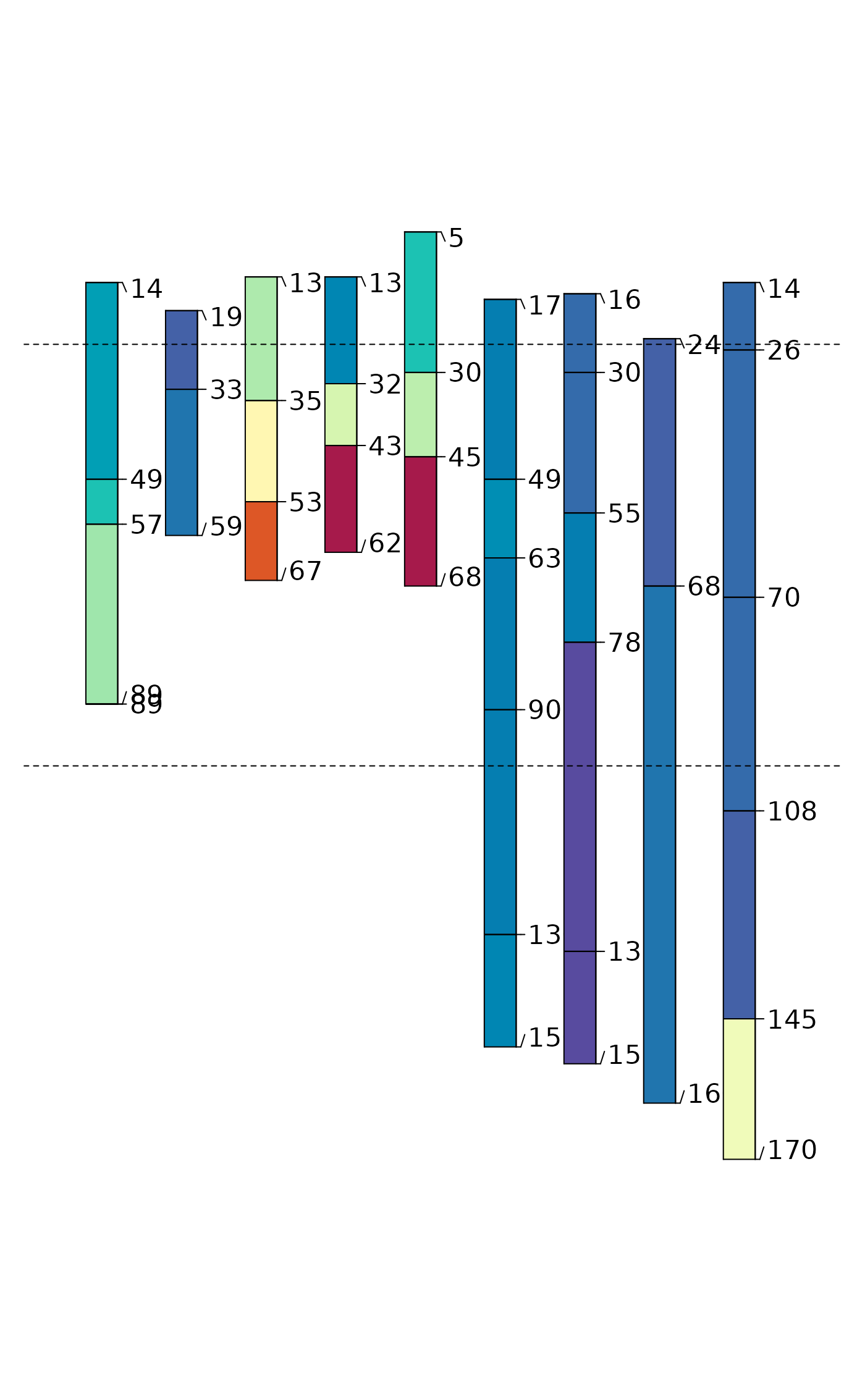## glom(..., truncate = TRUE)

p2 <- glom(sp1, 25, 150, truncate = TRUE)

# 28 horizons
nrow(p2)
#>  32

# inspect graphically
par(mar = c(1,1,3,1))
plot(p2, color = "prop", max.depth = 200)
#> [P001:4] horizon with top == bottom, cannot fix horizon depth overlap
#>  consider using repairMissingHzDepths()
abline(h = c(25, 100), lty = 2)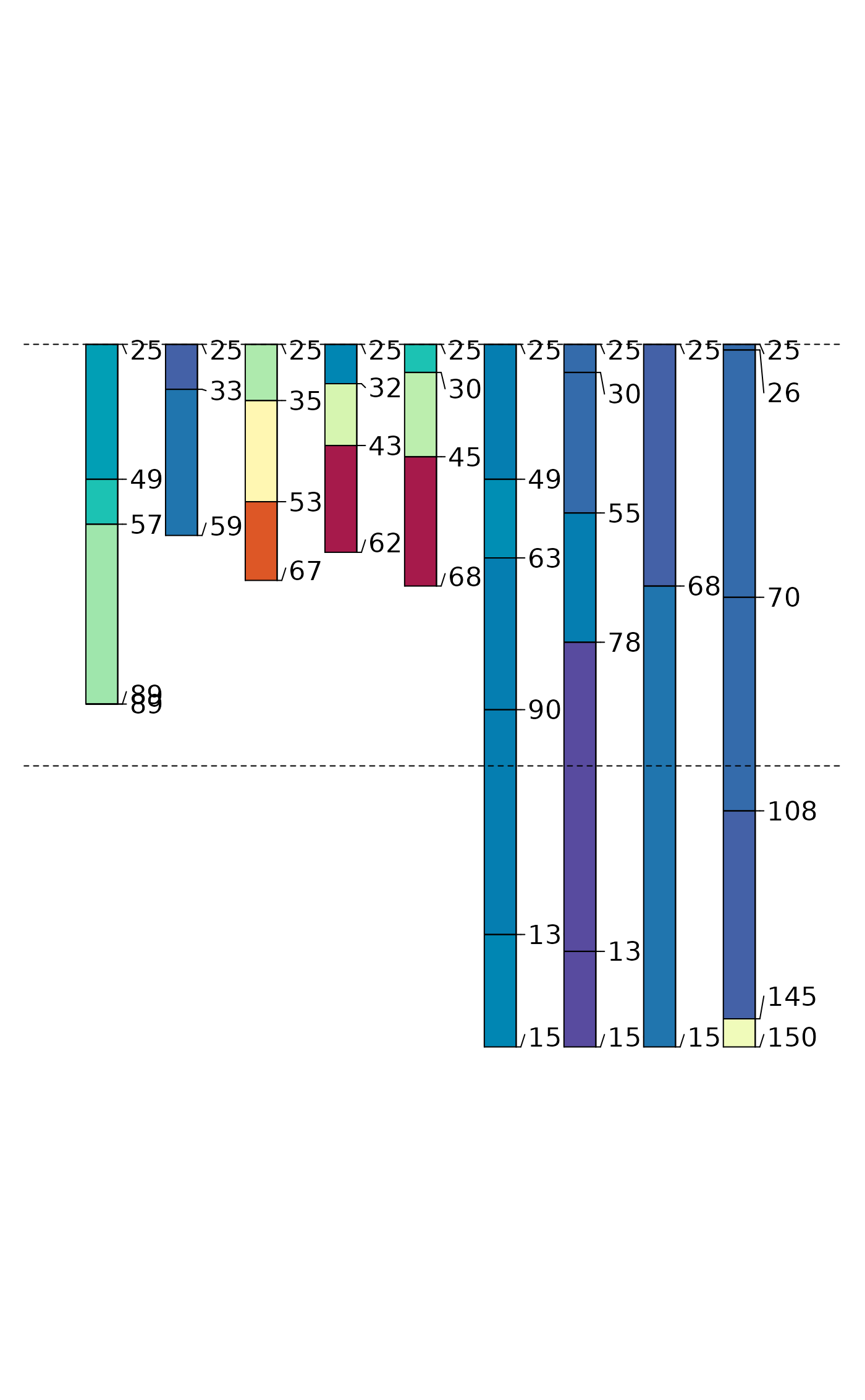## glom(..., truncate = TRUE, invert = TRUE)

p3 <- glom(sp1, 25, 150, truncate = TRUE, invert = TRUE)

# 45 horizons
nrow(p3)
#>  40

# inspect graphically
par(mar = c(1,1,3,1))
plot(p3, color = "prop", max.depth = 200)
abline(h = c(25, 100), lty = 2)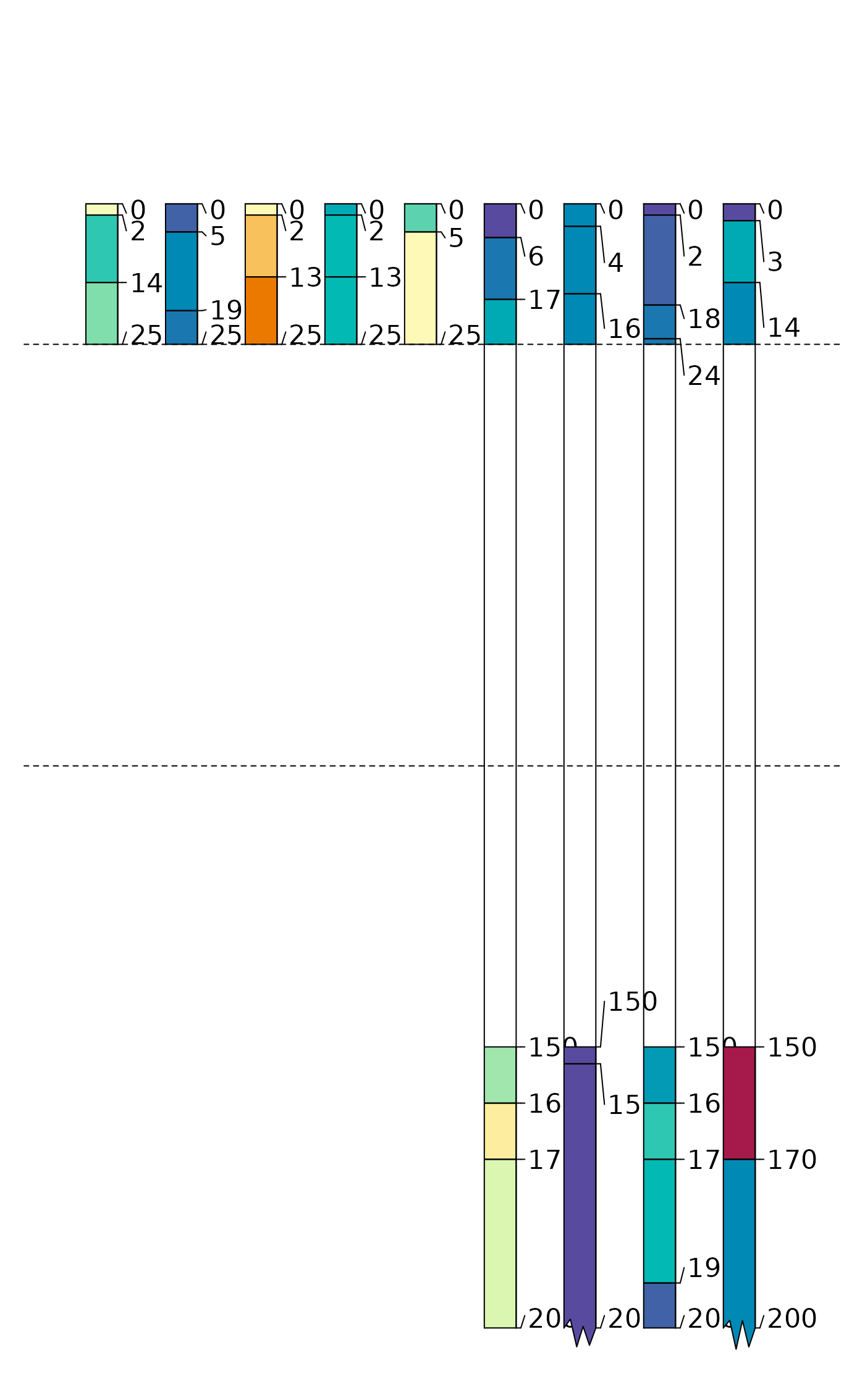## profile-specific interval, using expressions evaluated within sp1@site

# calculate some new site-level variables containing target interval
sp1$glom_top <- (1:9) * 10 sp1$glom_bottom <- 10 + sp1\$glom_top

# glom evaluates non-standard expressions using siteNames(sp1) column names
p4 <- glom(sp1, glom_top / 2, glom_bottom * 1.2, truncate = TRUE)

# inspect graphically
par(mar = c(1,1,3,1))
plot(p4, color = "prop", max.depth = 200)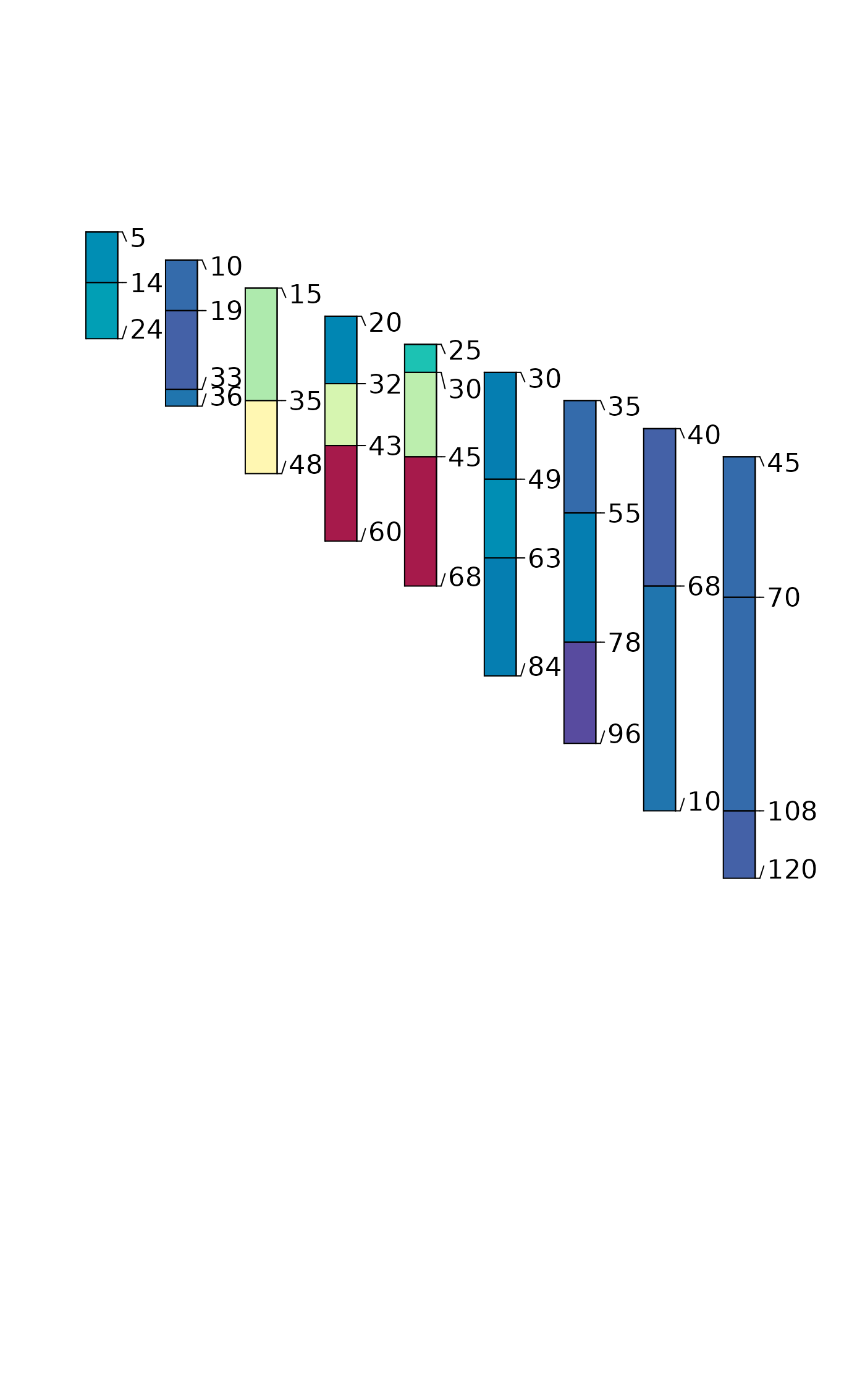data("sp3")

# promote to SPC
depths(sp3) <- id ~ top + bottom

### TRUNCATE all profiles in sp3 to [0,25]

# set up plot parameters
par(mfrow=c(2,1), mar=c(0,0,0,0))

# full profiles
plot(sp3)

# trunc'd profiles
plot(trunc(sp3, 0, 25))